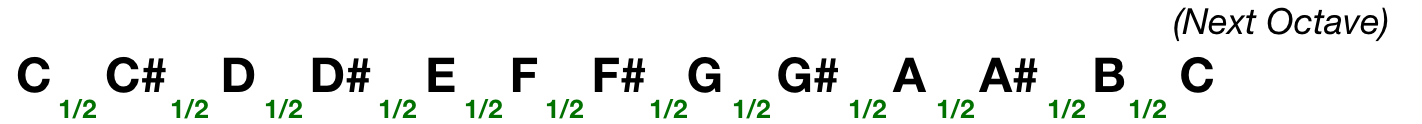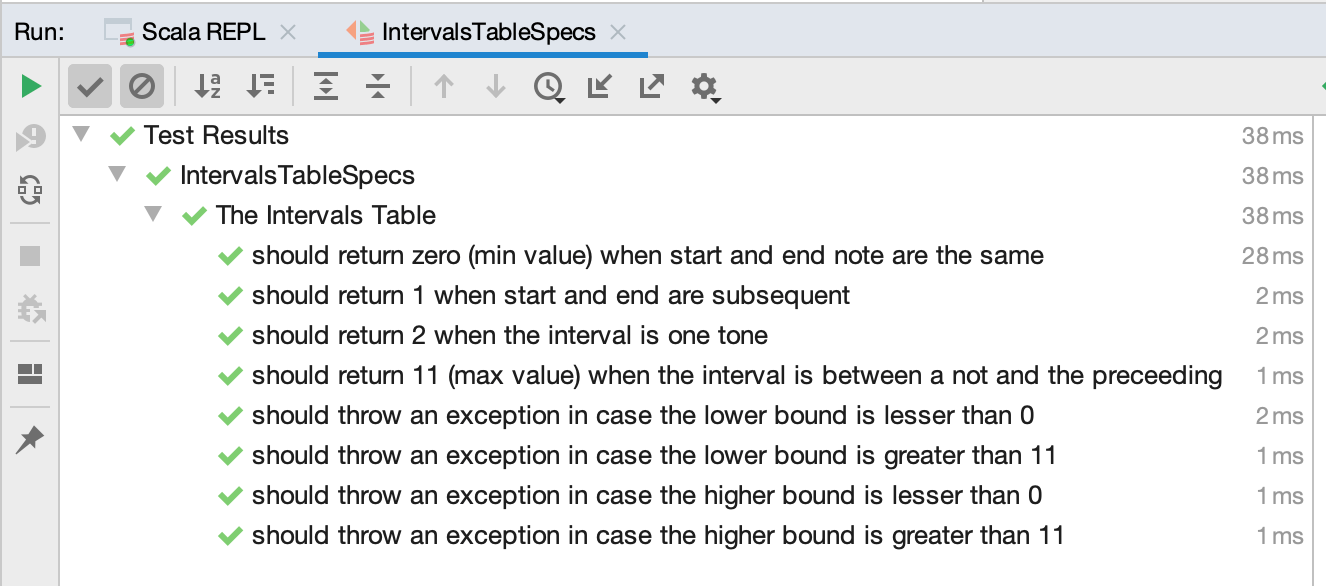# Music Information Retrieval: the Intervals Table

In this post we describe what is an Intervals Table and how to build it using a Behaviour-Driven-Development (BDD) approach.

The Intervals Table has been introduced in our London Information Retrieval meetup, in October 2019 (here the slides): it is a core component in the Cover Song Detection approach we are investigating in. Actually, it’s the “Interval” which represents a central concept in that approach, because it allows to think in terms of “relativeness” without caring about absolute frequencies or pitches.

## What is an Interval?

The music theory defines an interval as the difference in pitch between two sounds.

An interval can be horizontal (or linear or melodic), if it represents the distance between two successively sounding tones, and vertical or harmonic if it pertains to simultaneously sounding tones, such as in a chord.In Western music, an interval represent the distance between two notes of a diatonic scale. The smallest of these intervals is a semitone.

The diatonic scale is a musical scale that has

• seven pitches per octave
• five double semitones (a.k.a. tones) intervals
• two semitones intervals separated by two of three tones intervals

The C-Major scale is a perfect example of a diatonic scale (1/2 = semitone, 1 = Tone):In the scale above, we can say that

• the distance between C and D is 1 tone (or 2 semitones)
• the distance between D and G is 2 tones and a half (or 5 semitones)
• the distance between A and C is 1 tone and a half (or 3 semitones)

In Western music, the musical scale which has

• twelve pitches per octave
• twelve semitones intervals

is called chromatic scale. It lists all possible notes (again, in the Western Music):## The Intervals Table

The Intervals Table is a matrix which defines the possible intervals between each note of a chromatic scale

 C C# D D# E F F# G G# A A# B C 0 1 2 3 4 5 6 7 8 9 10 11 C# 11 0 1 2 3 4 5 6 7 8 9 10 D 10 11 0 1 2 3 4 5 6 7 8 9 D# 9 10 11 0 1 2 3 4 5 6 7 8 E 8 9 10 11 0 1 2 3 4 5 6 7 F 7 8 9 10 11 0 1 2 3 4 5 6 F# 6 7 8 9 10 11 0 1 2 3 4 5 G 5 6 7 8 9 10 11 0 1 2 3 4 G# 4 5 6 7 8 9 10 11 0 1 2 3 A 3 4 5 6 7 8 9 10 11 0 1 2 A# 2 3 4 5 6 7 8 9 10 11 0 1 B 1 2 3 4 5 6 7 8 9 10 11 0

Although in Music Theory each interval is denoted using a name (e.g. 0 = unison) the table uses the number of semitones for indicating the distance.

Using the Intervals Table we can say that:

• the distance between A and D# is 6 semitones
• the distance between B and B is 0 semitones (unison)

If we indicate the notes in the table using a 0-based position (e.g. C = 0, C# = 1, D = 2) the Intervals Table can be seen as a function that maps two notes (x, y) to a number which represents the distance in semitones.

In Scala

``````  def distanceBetween(x: Int, y: Int): Int =
if (y >= x) end - x else (11 - (x - 1)) + y``````

in Clojure

``````  (defn distance-between [x y]
(if (>= y x)
(-y x)
(+ (- 11 (- x 1)) y)))``````

an example of its usage:

``````> val table = new IntervalsTable()

> // 4 = E, 9 = A
> table.distanceBetween(4, 9)
res0: Int = 5

> table.distanceBetween(9, 4)
res0: Int = 7``````

The distanceBetween function computes the interval in semitones between two notes. Starting from there, it’s possible to create some high-level construct on top of it.

For instance, an overload version of the distanceBetween can compute the intervals between two vectors. The result in this case is another vector where each element represents the distance between the nth elements of the source vectors:

``````scala> val table = new IntervalsTable()
scala> table: io.sease.ra.IntervalsTable@16c5b50a

// Vector #1 = (C, E, G)
scala> val v1 = List(0, 4, 7)
v1: List[Int] = List(0, 4, 7)

// Vector #2 = (E, G, B)
scala> val v2 = List(4,7,11)
v2: List[Int] = List(4, 7, 11)

scala> table.distanceBetween(v1, v2)
res0: Seq[Int] = List(4, 3, 4)``````

Moving forward, at higher level the IntervalsTable should provide a function for computing the distances between the adjacent vectors in a matrix:

``````Input Matrix (3 x 4):

0  4  3  1
4  7  9  5
7  11 10 10

=

Distance between n1 and n2

0 - 4   =  4
4 - 7   =  7
7 - 11  =  11

Distance between n2 and n3
4  - 3  =  11
7  - 9  =  2
11 - 10 =  11

Distance between n3 and n4
3  - 1  =  10
9  - 5  =  8
10 - 10 =  0

Output matrix (3 x 3):

4  11  10
7  2   8
11 11  0``````

## The implementation

Instead of starting from the IntervalsTable class, let’s first define the behaviour we want to achieve:

The Intervals Table

• should return 0 when the start and end note is the same
• should return 1 when start and end notes are subsequent
• should return 2 if the interval is one tone (e.g 2 semitones)
• should return 11 when the interval is between a note and the preceding
• should throw an exception in case the lower bound is lesser than 0
• should throw an exception in case the lower bound is greater than 11
• should throw an exception in case the higher bound is lesser than 0
• should throw an exception in case the higher bound is greater than 11

Scala allows to translate of those assertions directly in test methods (sorry for the horizontal scrollbar, you can find the same code here):

```class IntervalsTableSpecs extends FlatSpec {

"The Intervals Table" should "return 0 (min value) when start and end note are the same" in {
val pipeline = new IntervalsTable()
0 to 11 by 1 foreach(idx => assert(pipeline.distanceBetween(idx, idx) == 0))
}

it should "return 1 when start and end are subsequent" in {
val pipeline = new IntervalsTable()

0 to 11 by 1 foreach(idx => assert(pipeline.distanceBetween(idx, if (idx == 11) 0 else idx + 1) == 1))
11 to 0 by -1 foreach(idx => assert(pipeline.distanceBetween(idx, if (idx == 11) 0 else idx + 1) == 1))
}

it should "return 2 when the interval is one tone" in {
val pipeline = new IntervalsTable()

0 to 10 by 2 foreach(idx => assert(pipeline.distanceBetween(idx, if (idx >= 10) (10 - idx) else idx + 2) == 2))
11 to 0 by -2 foreach(idx => assert(pipeline.distanceBetween(idx, if (idx >= 10) (11 - idx) + 1 else idx + 2) == 2))
}

it should "return 11 (max value) when the interval is between a note and the preceding" in {
val pipeline = new IntervalsTable()
0 to 10 by 2 foreach(idx => assert(pipeline.distanceBetween(idx, if (idx == 0) 11 else idx - 1) == 11))
}

it should "throw an exception in case the lower bound is lesser than 0" in {
val pipeline = new IntervalsTable()

assertThrows[IllegalArgumentException] {
pipeline.distanceBetween(-1, 8);
}
}

it should "throw an exception in case the lower bound is greater than 11" in {
val pipeline = new IntervalsTable()

assertThrows[IllegalArgumentException] {
pipeline.distanceBetween(12, 8);
}
}

it should "throw an exception in case the higher bound is lesser than 0" in {
val pipeline = new IntervalsTable()

assertThrows[IllegalArgumentException] {
pipeline.distanceBetween(8, -1);
}
}

it should "throw an exception in case the higher bound is greater than 11" in {
val pipeline = new IntervalsTable()

assertThrows[IllegalArgumentException] {
pipeline.distanceBetween(8, 12);
}
}
}```

And finally, here is the IntervalsTable class:

``````class IntervalsTable {

/**
* Returns the distance (in semitones) between
* the given start and end note.
*
* @param start the lower bound note.
* @param end the higher bound note.
* @return the distance between the given start and end note.
*/
def distanceBetween(start: Int, end: Int): Int = {
require(start >= 0 && start < 12)
require(end >= 0 && end < 12)
if (end >= start) end - start else (11 - (start - 1)) + end
}

/**
* Given two equal-sized vectors t1 and t2,
* returns a vector consisting of the distance
* between notes at the same index.
* In the following example t1 is a CMaj chord, t2 is Em:
*
* t1 t2        res
*
* 0  4   ==>    4
* 4  7   ==>    3
* 7  11  ==>    4
*
* @param t1 note vector at time t1
* @param t2 note vector at time t2
* @return a distance vector between t1 and t2
*/
def distanceBetween(t1: Seq[Int], t2: Seq[Int]): Seq[Int] = {
assert(t1.length == t2.length)
(t1, t2).zipped.map(distanceBetween)
}
}``````

What else? Let’s execute the tests and make sure the table meets the expected behaviour:

``````> sbt test

...
[info] Done compiling.
[info] Formatting 1 Scala source in shared_module:test ...
[info] Reformatted 1 Scala source in shared_module:test

...

[info] Done compiling

...

[info] IntervalsTableSpecs:
[info] The Intervals Table
[info] - should return 0 (min value) when start and end note are the same
[info] - should return 1 when start and end are subsequent
[info] - should return 2 when the interval is one tone
[info] - should return 11 when the interval is between a note and the preceding
[info] - should throw an exception in case the lower bound is lesser than 0
[info] - should throw an exception in case the lower bound is greater than 11
[info] - should throw an exception in case the higher bound is lesser than 0
[info] - should throw an exception in case the higher bound is greater than 11

...

[info] Total number of tests run: 8
[info] Suites: completed 1, aborted 0
[info] Tests: succeeded 8, failed 0, canceled 0, ignored 0, pending 0
[info] All tests passed.``````

the same execution, in IntelliJ:to be continued in the next episode…

// our service

## Shameless plug for our training and services!

Did I mention we do Apache Solr Beginner and Elasticsearch Beginer training?
We also provide consulting on these topics, get in touch if you want to bring your search engine to the next level!

// STAY ALWAYS UP TO DATE